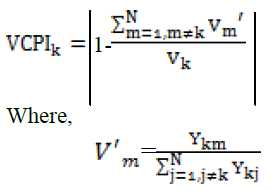## WEAK BUS IDENTIFICATION

A pilot bus or weak bus is defined as a bus that, when supported, improves the voltage profile at all the buses and also ensures additional security to the system, in terms of increased loading margin. Usually, placing adequate reactive power support at the weakest bus enhances static voltage stability margins. The bus which is close to experiencing voltage collapse is the weakest bus. Changes in voltage at each bus for a given change in system load are available from the tangent vector, which can be readily obtained from the voltage collapse proximity index prediction index (VCPI) is calculated at every bus. The value of the index determines the proximity to voltage collapse at a bus.

The technique is derived from the basic power flow equation, which is applicable to any number of buses in a system. The power flow equations are solved by Newton Raphson’s method, which creates a partial matrix. By setting the determinant of the matrix to zero, the index at bus k is written as follows:Vm is the phasor voltage at bus m

Vk is the phasor voltage at bus k

Ykm is the admittance between bus k and m

Ykj is the admittance between bus k and j

k is the monitoring bus

m is the other bus connected to the bus

N is the bus set of the system.

By finding the VCPI index we can find the weak bus and the weakest bus. Thus for this weak bus and the weakest buses, we are implementing the FACT device like STATCOM and TCSC.